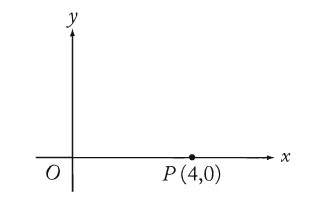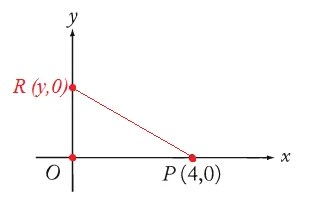Breaking News

# GMAT Problem Solving Questions with Answers – Test No. 1

GMAT Problem Solving Questions with Answers – Practice Test No. 1 for those who want to test their GMAT – Graduate Management Admission Test skills. GMAT is a standardized test used for the evaluation of students seeking admission in BBA, MBA, and post-graduate studies in Pakistan or abroad. Management studies provide you with the skills, preparation, and credentials you need to accelerate your career growth. MBA is the most popular degree program in Pakistan. LUMS and IBA MBA programs are the most applied in Pakistan.

Each quiz question in this course is made up of proven interesting researched concepts that test your awareness and grasp of the subject. Detailed feedback for the quiz answers is provided too.

INSTRUCTIONS:

• This Quiz is related to GMAT Problem Solving Questions with Answers – Practice Test No. 1 in this series
• The Quiz based on the Topic, “GMAT Problem Solving Questions
• If you have good understanding about the subject, then you can attempt it
• There will be 10 Multiple Choice Questions (MCQs) in this test
• Questions will be randomly changed every time you start this test
• You should practice more and more to get high marks
• You can retake this test as many time as you like
• If you feel that any Incorrect Answer to a Question, simply Comment us about the Question.

#### In the rectangular coordinate system above, if point R (not shown) lies on the positive y-axis and the area of triangle ORP is 12, what is the y-coordinate of point R?Correct! Wrong!

#### In the rectangular coordinate system above, if point R (not shown) lies on the positive y-axis and the area of triangle ORP is 12, what is the y-coordinate of point R?Correct! Wrong!

The area of triangle OPR is 1/2*base*height=1/2*OP*OR=1/2*4*OR=12 --> OR=6.

Correct! Wrong!

Correct! Wrong!

#### A certain pair of used shoes can be repaired for \$12.50 and will last for 1 year. A pair of the same kind of shoes can be purchased new for \$28.00 and will last for 2 years. The average cost per year of the new shoes is what percent greater than the cost of repairing the used shoes?

Correct! Wrong!

28 / 2 = 14 14 - 12.50 = 1.50 1.50 / 12.50 = .12 = 12% Answer choice C

#### In the figure above, if PQRS is a parallelogram, then y - x =Correct! Wrong!

In parallelogram corresponding angles are equal and consecutive angles are supplementary (supplementary angles are two angles that add up to 180°), thus: 140=2y140=2y --> y=70y=70; 2y+x=1802y+x=180 --> 140+x=180140+x=180 --> x=40x=40; Therefore, y−x=70−40=30y−x=70−40=30. Answer: A.

#### If a = 105 and a^3 = 21 × 25 × 45 × b, what is the value of b?

Correct! Wrong!

Explanation This question calls for some prime factorization a = 105 = (3)(5)(7) So, a³ = [(3)(5)(7)]³ = (3)(3)(3)(5)(5)(5)(7)(7)(7) We're told that a³ = 21 × 25 × 45 × b Take: a³ = (3)(3)(3)(5)(5)(5)(7)(7)(7) Rewrite as: a³ = (3)(3)(3)(5)(5)(5)(7)(7)(7) = (3)(3)(21)(5)(5)(5)(7)(7) Now rewrite as: a³ = (3)(3)(21)(5)(5)(5)(7)(7) = (3)(3)(21)(25)(5)(7)(7) Now rewrite as: a³ = (3)(3)(21)(25)(9)(7)(7) = (21)(25)(45)(7)(7) If 21 × 25 × 45 × b = (21)(25)(45)(7)(7), then b = (7)(7) = 49

#### Company Q plans to make a new product next year and sell each unit of this new product at a selling price of \$2. The variable costs per unit in each production run are estimated to be 40% of the selling price, and the fixed costs for each production run are estimated to be \$5,040. Based on these estimated costs, how many units of the new product will Company Q need to make and sell in order for their revenue to equal their total costs for each production run?

Correct! Wrong!

Explanation The variable costs per unit = 0.4*2 = \$0.8. So, we are left with \$1.2. To break even with \$5,040 of fixed costs, \$5,040/1.2 = \$5,040*5/6 = 4,200 units should be made. Answer: A.

#### What is the combined area, in square inches, of the front and back of a rectangular sheet of paper measuring 8.5 inches by 11 inches?

Correct! Wrong!

Explanation Im not sure what does front and back area means, do we have to multiply by 2. front side area of rectangle : 11*8.5: 93.5 in2 back side area of rectangle : 93.5+93.5: 187 in2 option E ================================================================= Area of a rectangle = l x b = 11 x 8.5 = 93.5 Front and Back side = 93.5+93.5 = 187 the answer is E. =================================================================== Area = (base)(height) We want the combined area of BOTH sides (front and back) So, the combined area = (8.5)(11) + (8.5)(11) = (17)(11) = 187 Answer: E

#### A certain population of bacteria doubles every 10 minutes. If the number of bacteria in the population initially was 10^4, what was the number in the population 1 hour later?

Correct! Wrong!

Explanation Let's list some populations and look for a pattern Initial population: 10⁴ 10 minutes later: (10⁴)(2) 20 minutes later: (10⁴)(2)(2) 30 minutes later: (10⁴)(2)(2)(2) 40 minutes later: (10⁴)(2)(2)(2)(2) 50 minutes later: (10⁴)(2)(2)(2)(2)(2) 60 minutes later: (10⁴)(2)(2)(2)(2)(2)(2) = (10⁴)(2⁶) Answer: C

GMAT Problem Solving MCQs with Answers Test # 1

GMAT Problem Solving MCQs with Answers Test # 2

GMAT Problem Solving MCQs with Answers Test # 3

GMAT Problem Solving MCQs with Answers Test # 4

GMAT Problem Solving MCQs with Answers Test # 5

NTS Preparation for NAT Test

## Bank Reconciliation Statement – MCQs with Answers – Quiz 2

Bank Reconciliation Statement – MCQs with Answers Bank Reconciliation Statement is a very helpful tool …